## Landau Constant

N.B. A detailed on-line essay by S. Finch was the starting point for this entry.

Let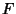be the set of Complex analytic functions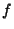defined on an open region containing the closure of the unit disk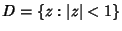satisfyingand. For eachin, let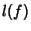be the Supremum of all numberssuch that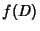contains a disk of radius. ThenThis constant is called the Landau constant, or the Bloch-Landau Constant. Robinson (1938, unpublished) and Rademacher (1943) derived the bounds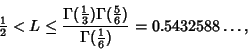whereis the Gamma Function, and conjectured that the second inequality is actually an equality,Finch, S. Favorite Mathematical Constants.'' http://www.mathsoft.com/asolve/constant/bloch/bloch.html
Rademacher, H. On the Bloch-Landau Constant.'' Amer. J. Math. 65, 387-390, 1943.